# 10+2 Data Science Methods that Every Data Scientist Should Know in 2016

Two years ago, I published a book -- written in Japanese so I'm afraid most of the readers can't read it :'(

Actually this book was written as a summary of 10 major data science methods. But as two years have gone, the content of the book is now out-of-date; obviously it needs further update, including some more advances in statistics and machine learning. Below is a list of the 10+2 methods that I believe every data scientist must know in 2016.

1. Statistical Hypothesis Testing (t-test, chi-squared test & ANOVA)
2. Multiple Regression (Linear Models)
3. General Linear Models (GLM: Logistic Regression, Poisson Regression)
4. Random Forest
5. Xgboost (eXtreme Gradient Boosted Trees)
6. Deep Learning
7. Bayesian Modeling with MCMC
8. word2vec
9. K-means Clustering
10. Graph Theory & Network Analysis
• (A1) Latent Dirichlet Allocation & Topic Modeling
• (A2) Factorization (SVD, NMF)

The first 10 methods are the ones I know well and indeed I'm running in my daily works, but I've never tried the last 2 methods by my own hand for actual business and they've been run by my colleagues in my previous job, although I'm also familiar with them as an operation manager. So the former includes R or Python scripts to run as practical examples, while the latter only includes ordinary examples provided by help sources. Some of them require gcc / clang compiler, or Java runtime environment such as H2O. OK, let's go.

Disclaimer

• This post gives you a 'perspective' for those who want to overview all of the methods; there may be some less strict and/or incorrect description, and it was not supposed to provide any knowledge on implementation from scratch.
• Please search how to install packages, libraries or other build environment by yourself.
• Any comments or critiques on any incorrect points in this post are welcome.

### Statistical Hypothesis Testing (t-test, chi-squared test & ANOVA)

I think this is one of the most popular statistical methods despite its long history, convention and traditionally frequenstic. This method is used for comparing something with the other, such as A/B testing, when not only difference itself but also its credibility is important. Actually rather statistical (multivariate) modeling than merely hypothesis testing works well because of rich representation of statistical modeling, but hypothesis testing is still popular in a lot of business scenes. Here we see 3 methods.

#### t-test

In general, t-test is used when you want to compare "mean" values between 2 groups and you have unaggregated raw datasets. An example below is to compare latencies of a certain query between 2 DBs and to clarify which DB is faster.

> d<-read.csv('https://raw.githubusercontent.com/ozt-ca/tjo.hatenablog.samples/master/r_samples/public_lib/DM_sampledata/ch3_2_2.txt',header=T,sep=' ')
DB1      DB2
1 0.9477293 2.465692
2 1.4046824 2.132022
3 1.4064391 2.599804
4 1.8396669 2.366184
5 1.3265343 1.804903
6 2.3114898 2.449027
> boxplot(d) # Plotting a box plot
> t.test(d$DB1,d$DB2) # t.test() function for t-test

Welch Two Sample t-test

data:  d$DB1 and d$DB2
t = -3.9165, df = 22.914, p-value = 0.0006957
alternative hypothesis: true difference in means is not equal to 0
95 percent confidence interval:
-1.0998402 -0.3394647
sample estimates:
mean of x mean of y
1.575080  2.294733
# Welch test, a testing without any assumption of homogenous variance, is automatically applied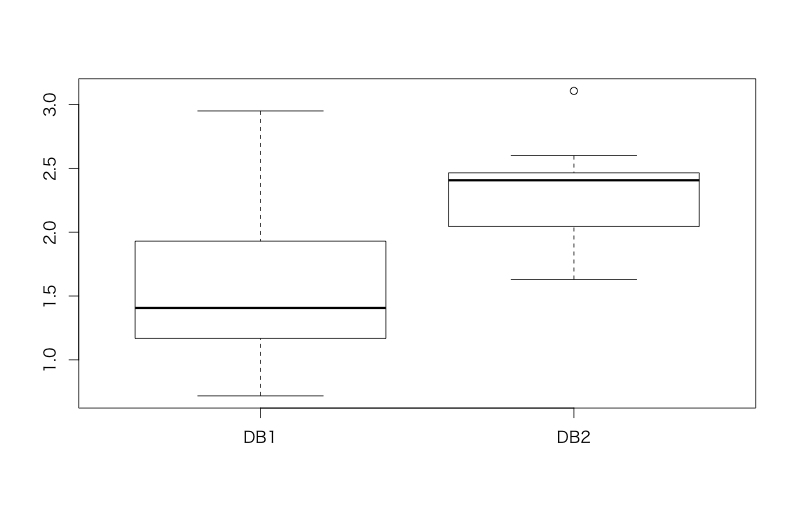The result p < 0.05 allows us to conclude that DB1 is faster than DB2.*1

#### Chi-squared test

This is a method for comparing "ratio" between conditions, e.g. conversion rate (CVR). For example, imagine you modified possible conversion paths in your smartphone app and then you saw the count of CV as below:

CV non CV
Before 25 117
After 16 32

In the case of "tabulated" datasets like this example, we cannot compare the "ratio" with considering any kinds of variability computed from raw and unaggregated datasets like t-test. Instead, we can run chi-squared test (test of independency) that compares whether both datasets are generated from a unique and identical distribution.

> d<-matrix(c(25,117,16,32),ncol=2,byrow=T)
> chisq.test(d) # chisq.test() for chi-squared test

Pearson's Chi-squared test with Yates' continuity correction

data:  d
X-squared = 4.3556, df = 1, p-value = 0.03689


We got p < 0.05 and can conclude that your modification significantly increases CV. FYI, when you have a series of results from multiple chi-squared test with the same intervention in distinct datasets, you can integrate them with Cochran-Mantel-Haenszel test. Of course, in case of t-test, you can also integrate with Rosenthal's method. Such meta-analysis techniques are very useful so please check them by yourself. :)

#### ANOVA (Analysis of Variance)

In principle, this method is an integrated version of t-test on more than 2 datasets and used when you want to compare means across more than 2 datasets with more than 1 intervention. This is a variant of multivariate analysis such as multiple regression (linear models). In particular ANOVA says whether there is a "main effect" and "interaction" of/between interventions (explanatory variables), and those are almost identical to coefficients in multiple regression. If you are interested in only whether there is any effect of interventions, ANOVA is the best; but if you are interested in both magnitude and direction of effects, you should use multiple regression.

Imagine you were selling 2 kinds of products at a department store in person: during 4 days, you were changing 2 kinds of promotion and now you want to know whether the type of promotion varies its revenue. Here we assume that variable 'pr' means which kind of promotion was used, 'category' means the category of product and 'cnt' means the revenue (dependent variable). You can compute an ANOVA model in R as below.

> d<-data.frame(cnt=c(210,435,130,720,320,470,250,380,290,505,180,320,310,390,410,510),pr=c(rep(c('F','Y'),8)),category=rep(c('a','a','b','b'),4))
> d.aov<-aov(cnt~.^2,d) # aov() function for ANOVA
> summary(d.aov)
Df Sum Sq Mean Sq F value  Pr(>F)
pr           1 166056  166056  12.984 0.00362 **
category     1     56      56   0.004 0.94822
pr:category  1   5256    5256   0.411 0.53353
Residuals   12 153475   12790
---
Signif. codes:  0 ‘***’ 0.001 ‘**’ 0.01 ‘*’ 0.05 ‘.’ 0.1 ‘ ’ 1


We can conclude that the promotion has a 'main effect' which means the promotion significantly increase or decrease revenue. On the other hand, there is either no difference between the categories or no interaction between the category and the promotion.

#### Other hypothesis testing

We are also using other testing such as F-test, rank-sum test (Wilcoxon / Mann-Whitney test) and even we have to be aware of difference between parametric tests (requiring assumption of distribution of datasets and its shape) and nonparametric tests (requires no assumption), but all of them are a little advanced issues so I won't explain them here.

### Multiple Regression (Linear Models)

I think this is one of the most basic methods in data science, but I feel it's still not so popular in practical business analytics although linear models are known as very easy methods in machine learning community and widely used for such machine learning systems even for business.

Below is an example of revenue of a beer brand in a certain district. Let's build a model for "Revenue" (of the beer per day) as a dependent variable with "CM" (a volume of TVCM per day), "Temp" (air temperature) and "Firework" (categorical variable indicating whether there were any fireworks show in the district) as independent variables.

> d<-read.csv('https://raw.githubusercontent.com/ozt-ca/tjo.hatenablog.samples/master/r_samples/public_lib/DM_sampledata/ch4_3_2.txt',header=T,sep=' ')
Revenue  CM Temp Firework
1 47.14347 141   31        2
2 36.92363 144   23        1
3 38.92102 155   32        0
4 40.46434 130   28        0
5 51.60783 161   37        0
6 32.87875 154   27        0
> d.lm<-lm(Revenue~.,d) # lm() function for linear models
> summary(d.lm)

Call:
lm(formula = Revenue ~ ., data = d)

Residuals:
Min     1Q Median     3Q    Max
-6.028 -3.038 -0.009  2.097  8.141

Coefficients:
Estimate Std. Error t value Pr(>|t|)
(Intercept) 17.23377   12.40527   1.389  0.17655
CM          -0.04284    0.07768  -0.551  0.58602
Temp         0.98716    0.17945   5.501    9e-06 ***
Firework     3.18159    0.95993   3.314  0.00271 **
---
Signif. codes:  0 ‘***’ 0.001 ‘**’ 0.01 ‘*’ 0.05 ‘.’ 0.1 ‘ ’ 1

Residual standard error: 3.981 on 26 degrees of freedom
Multiple R-squared:  0.6264,	Adjusted R-squared:  0.5833
F-statistic: 14.53 on 3 and 26 DF,  p-value: 9.342e-06

# Plotting
> matplot(cbind(d$Revenue,predict(d.lm,newdata=d[,-1])),type='l',lwd=c(2,3),lty=1,col=c(1,2)) > legend('topleft',legend=c('Data','Predicted'),lwd=c(2,3),lty=1,col=c(1,2),ncol=1)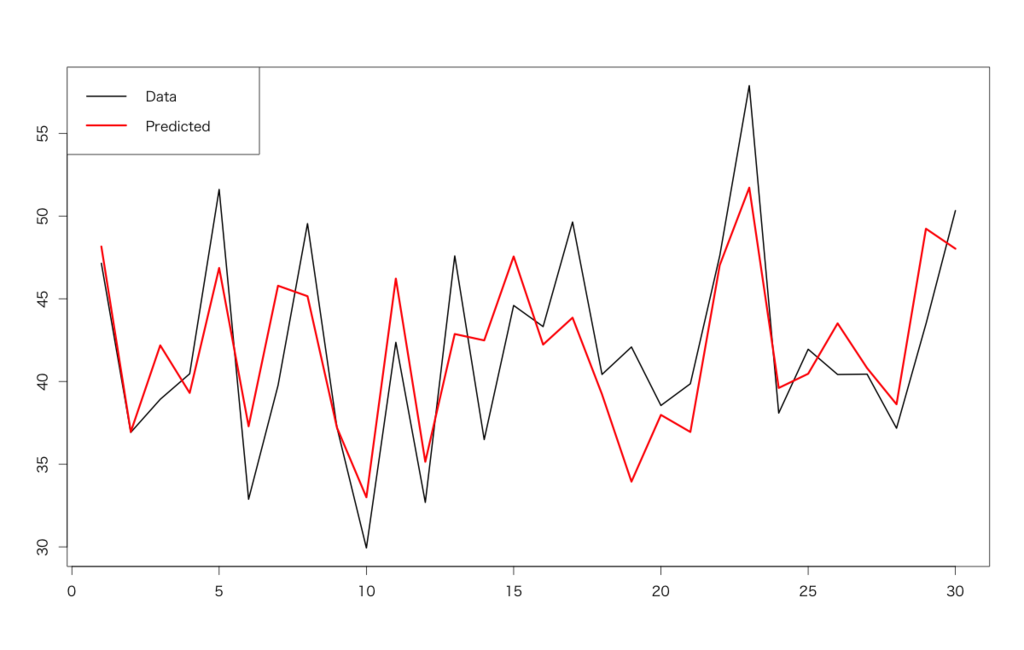We can conclude that temperature and whether there were fireworks shows on each day are important. Of course if we can obtain any future values of the independent variables (indeed TVCM are planned in advance and temperature are officially forecasted), we can forecast future revenue with predict() method.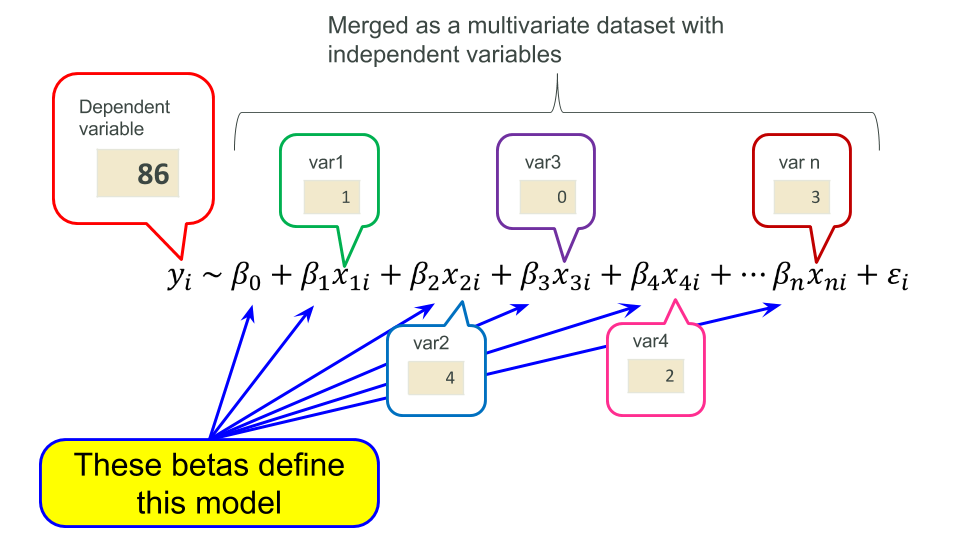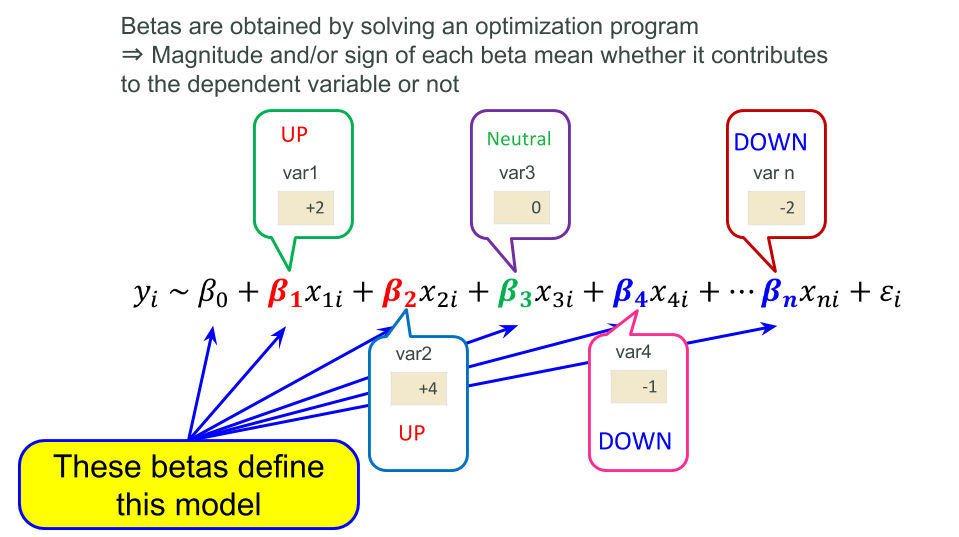In short, linear modeling is a method representing a dependent variable with summation of products of independent variables and coefficients (parameters) that are estimated with optimization programming. This idea is common across most of statistical modeling and even machine learning (in particular logistic regression and/or many online learning algorithms), so please keep them in mind. (Notice: a lot of textbooks refer linear models as a 'basic' model of machine learning. In many implementations parameters are directly estimated by matrix calculation, but I recommend to implement an algorithm with the gradient descent in Python or other script languages in order to understand what it works) ### General Linear Models (GLM: logistic regression, Poisson regression) I think GLM is at the border between statistics and machine learning, and also one of the most interesting matter in statistical modeling. It is almost the same as linear models, but in GLM its dependent variable is NOT assumed to be normally distributed; we have to care about how it should be distributed, e.g. with Poisson distribution, Negative-binomial distribution, etc. #### Logistic Regression This is a binary classification and also a basic but important method in machine learning. Its dependent variable is distributed with binomial distribution. Below is an example from the chapter 6 of my textbook, in which "cv" is the dependent variable as the count of conversion of a certain e-commerce service and "d21-d26" are the independent variables as distinct promotion pages. We are supposed to clarify which promotion page contributes to CV. > d<-read.csv('https://raw.githubusercontent.com/ozt-ca/tjo.hatenablog.samples/master/r_samples/public_lib/DM_sampledata/ch6_4_2.txt',header=T,sep=' ') > d$cv<-as.factor(d$cv) # Casting cv to categorical > d.glm<-glm(cv~.,d,family=binomial) # "family" argument handles what kind of distribution should be used > summary(d.glm) Call: glm(formula = cv ~ ., family = binomial, data = d) Deviance Residuals: Min 1Q Median 3Q Max -2.3793 -0.3138 -0.2614 0.4173 2.4641 Coefficients: Estimate Std. Error z value Pr(>|z|) (Intercept) -1.0120 0.9950 -1.017 0.3091 d21 2.0566 0.8678 2.370 0.0178 * d22 -1.7610 0.7464 -2.359 0.0183 * d23 -0.2136 0.6131 -0.348 0.7276 d24 0.2994 0.8368 0.358 0.7205 d25 -0.3726 0.6064 -0.614 0.5390 d26 1.4258 0.6408 2.225 0.0261 * --- Signif. codes: 0 ‘***’ 0.001 ‘**’ 0.01 ‘*’ 0.05 ‘.’ 0.1 ‘ ’ 1 (Dispersion parameter for binomial family taken to be 1) Null deviance: 173.279 on 124 degrees of freedom Residual deviance: 77.167 on 118 degrees of freedom AIC: 91.167 Number of Fisher Scoring iterations: 5  We can conclude that "d21" is the best and "d22" is the worst. Of course this model can forecast future dependent variable with predict() method and independent variables in the future. #### Poisson Regression In contrast to logistic regression, Poisson regression is rather statistical modeling than machine learning, used in the case that its dependent variable is distributed with Poisson distribution. You'll see a lot of definition and/or examples about Poisson distribution if you're Googling, but in principle it represents counts of some "rare" events. For example, a histogram as below means Poisson distribution.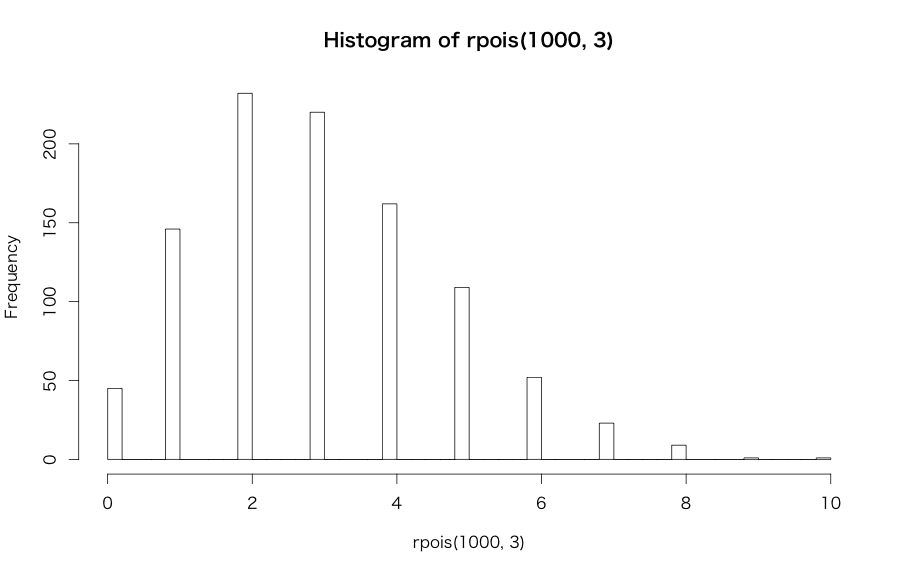In actual business scenes, the number of conversions per day against the number of page views at a certain web site is the most famous example distributed as Poisson distribution*2. By the way, very unfortunately I have no good example for Poisson regression... so here let's use the original example raised in R help :( According to the help, this dataset is from "An Introduction to Generalized Linear Models" by Dobson in 1990. > ## Dobson (1990) Page 93: Randomized Controlled Trial : > counts <- c(18,17,15,20,10,20,25,13,12) > outcome <- gl(3,1,9) > treatment <- gl(3,3) > print(d.AD <- data.frame(treatment, outcome, counts)) treatment outcome counts 1 1 1 18 2 1 2 17 3 1 3 15 4 2 1 20 5 2 2 10 6 2 3 20 7 3 1 25 8 3 2 13 9 3 3 12 > glm.D93 <- glm(counts ~ outcome + treatment, family = poisson) # "family" argument should be "poisson" > summary(glm.D93) Call: glm(formula = counts ~ outcome + treatment, family = poisson) Deviance Residuals: 1 2 3 4 5 6 7 8 9 -0.67125 0.96272 -0.16965 -0.21999 -0.95552 1.04939 0.84715 -0.09167 -0.96656 Coefficients: Estimate Std. Error z value Pr(>|z|) (Intercept) 3.045e+00 1.709e-01 17.815 <2e-16 *** outcome2 -4.543e-01 2.022e-01 -2.247 0.0246 * outcome3 -2.930e-01 1.927e-01 -1.520 0.1285 treatment2 1.338e-15 2.000e-01 0.000 1.0000 treatment3 1.421e-15 2.000e-01 0.000 1.0000 --- Signif. codes: 0 ‘***’ 0.001 ‘**’ 0.01 ‘*’ 0.05 ‘.’ 0.1 ‘ ’ 1 (Dispersion parameter for poisson family taken to be 1) Null deviance: 10.5814 on 8 degrees of freedom Residual deviance: 5.1291 on 4 degrees of freedom AIC: 56.761 Number of Fisher Scoring iterations: 4 # AIC gives how the model is generalized and a ratio of Residual deviance and degrees of freedom indicates how the model is overdispersed. # If the ratio is too larger than 1, it's overdispersed > hist(counts,breaks=50)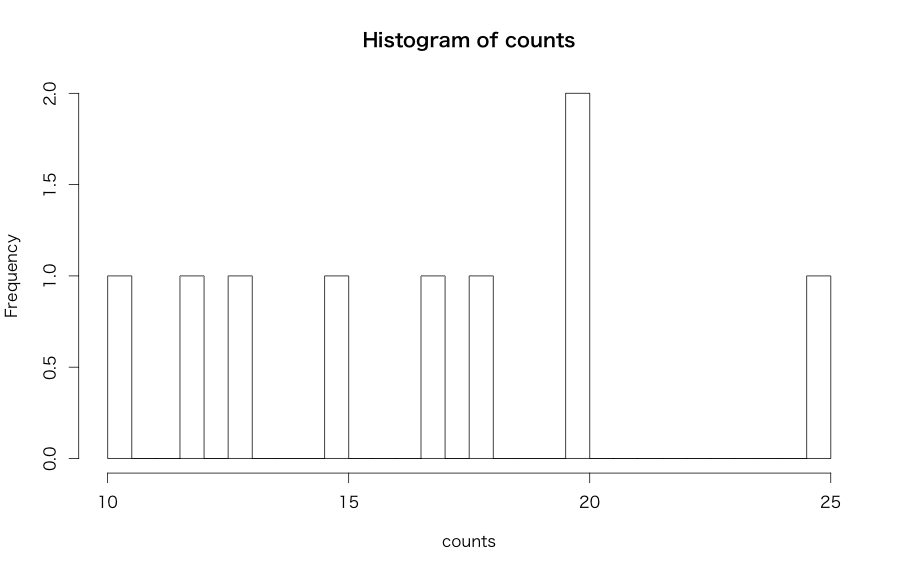The result shows outcome2 is important. In general, Poisson regression may not work well when dependent variable includes too many zeros (overdispersion), so in such a case you have to use negative-binomial regression instead of Poisson regression. {VGAM} package provides glm.nb() function for negative-binomial regression. #### Regularization / Penalization (L1 / L2 norm) Just in my opinion, but regularization / penalization is rather machine learning matter than statistical one. "Generalization" is a term representing to what extent a model fits not only training data but also test data. Regularization is one of the strong methods to generalize a model: in general, when you apply some generalization, parameters of a model will be estimated with a certain restriction in order to prevent them from overfitting. In particular, "splitting test dataset from training dataset" (cross validation) is very much important in machine learning. If not, obtained models may fit not only training data themselves but also a lot of "noises" with them. Cross validation has some variation, e.g. holdout, leave-one-out, k-folds, etc. Here I tried Lasso regression (L1-regularized logistic regression) onto a dataset names "Tennis", distributed by UC Irvine ML repository. Men's dataset is used for training and women's dataset is used for test as hold-out cross validation. Lasso regression is a method cutting unnecessary independent variables. > dm<-read.csv('https://github.com/ozt-ca/tjo.hatenablog.samples/raw/master/r_samples/public_lib/jp/exp_uci_datasets/tennis/men.txt',header=T,sep='\t') > dw<-read.csv('https://github.com/ozt-ca/tjo.hatenablog.samples/raw/master/r_samples/public_lib/jp/exp_uci_datasets/tennis/women.txt',header=T,sep='\t') > dm<-dm[,-c(1,2,16,17,18,19,20,21,34,35,36,37,38,39)] > dw<-dw[,-c(1,2,16,17,18,19,20,21,34,35,36,37,38,39)] # L1 regularization > library(glmnet) > dm.cv.glmnet<-cv.glmnet(as.matrix(dm[,-1]),as.matrix(dm[,1]),family="binomial",alpha=1) # alpha=1 for L1 regularization, alpha=0 for L2 regularization, and (0, 1) for elastic net # cv.glmnet() function optimizes a parameter with cross validation > plot(dm.cv.glmnet) > coef(dm.cv.glmnet,s=dm.cv.glmnet$lambda.min) # "s" argument requires the optimized parameter
25 x 1 sparse Matrix of class "dgCMatrix"
1
(Intercept)  3.533402e-01
FSP.1        3.805604e-02
FSW.1        1.179697e-01
SSP.1       -3.275595e-05
SSW.1        1.475791e-01
ACE.1        .
DBF.1       -8.934231e-02
WNR.1        3.628403e-02
UFE.1       -7.839983e-03
BPC.1        3.758665e-01
BPW.1        2.064167e-01
NPA.1        .
NPW.1        .
FSP.2       -2.924528e-02
FSW.2       -1.568441e-01
SSP.2        .
SSW.2       -1.324209e-01
ACE.2        1.233763e-02
DBF.2        4.032510e-02
WNR.2       -2.071361e-02
UFE.2       -6.114823e-06
BPC.2       -3.648171e-01
BPW.2       -1.985184e-01
NPA.2        .
NPW.2        1.340329e-02
> table(dw$Result,round(predict(dm.cv.glmnet,as.matrix(dw[,-1]),s=dm.cv.glmnet$lambda.min,type='response'),0))

0   1
0 215  12
1  18 207
> sum(diag(table(dw$Result,round(predict(dm.cv.glmnet,as.matrix(dw[,-1]),s=dm.cv.glmnet$lambda.min,type='response'),0))))/nrow(dw)
 0.9336283 # Accuracy 93.4 %

# Comparison: case of usual logistic regression
> dm.glm<-glm(Result~.,dm,family=binomial)
> table(dw$Result,round(predict(dm.glm,newdata=dw[,-1],type='response'))) 0 1 0 211 16 1 17 208 > sum(diag(table(dw$Result,round(predict(dm.glm,newdata=dw[,-1],type='response')))))/nrow(dw)
 0.9269912 # Accuracy 92.7 %


Indeed, L1-regularized logistic regression that cuts unnecessary independent variables is better than usual logistic regression in terms of hold-out accuracy. It means that more "generalization" is given by L1 regularization.

#### Other GLMs

Basically you can choose any kind of GLMs by choosing "family" argument in glm() function. Perhaps you may wonder which quasi-poisson or glm.nb should be used, but
you only have to learn more details every time you need more.

### Random Forest

From now on we're getting into typical machine learning techniques. The first one is Random Forest, which is widely used in product environment of various machine learning systems. It's one of the most famous implementation of "bagging" ensemble machine learning methods so now we see a lot of libraries or packages implementing random forest and they're widely used all over the world. In a previous post I argued about its detail.

Here I tried it onto the famous MNIST handwritten digit datasets to see how Random Forest works. Its original dataset is too large so I prepared a short version in which training dataset with 5,000 rows and test datasets with 1,000 rows on my GitHub repository.

> train<-read.csv('https://github.com/ozt-ca/tjo.hatenablog.samples/raw/master/r_samples/public_lib/jp/mnist_reproduced/short_prac_train.csv')
> train$label<-as.factor(train$label)
> test$label<-as.factor(test$label)

> library(randomForest)
> train.rf<-randomForest(label~.,train)
> table(test$label,predict(train.rf,newdata=test[,-1])) 0 1 2 3 4 5 6 7 8 9 0 96 0 0 0 0 0 3 0 1 0 1 0 99 0 0 0 0 0 0 1 0 2 0 0 96 1 1 0 1 1 0 0 3 0 0 2 87 0 4 1 1 3 2 4 0 0 0 0 96 0 1 0 0 3 5 1 2 0 1 0 94 2 0 0 0 6 0 0 1 0 1 2 95 0 1 0 7 0 2 0 0 1 0 0 93 0 4 8 0 0 1 0 0 0 0 0 99 0 9 0 0 0 0 2 1 0 1 0 96 > sum(diag(table(test$label,predict(train.rf,newdata=test[,-1]))))/nrow(test)
 0.951 # Accuracy 95.1 %


Random Forest demonstrated accuracy 95.1 % and this post regards it as a benchmark. Indeed Kaggle MNIST competition also shows an example script with the same {randomForest} CRAN package as their benchmark.

If you're interested, it's a good idea to draw MNIST handwritten digits on R. You can plot it as below.

> par(mfrow=c(3,4))
> for(i in 1:10){
+     image(t(apply(matrix(as.vector(as.matrix(train[(i-1)*500+50,-1])),ncol=28,nrow=28,byrow=T),2,rev)),col=grey(seq(0,1,length.out=256)))
+ }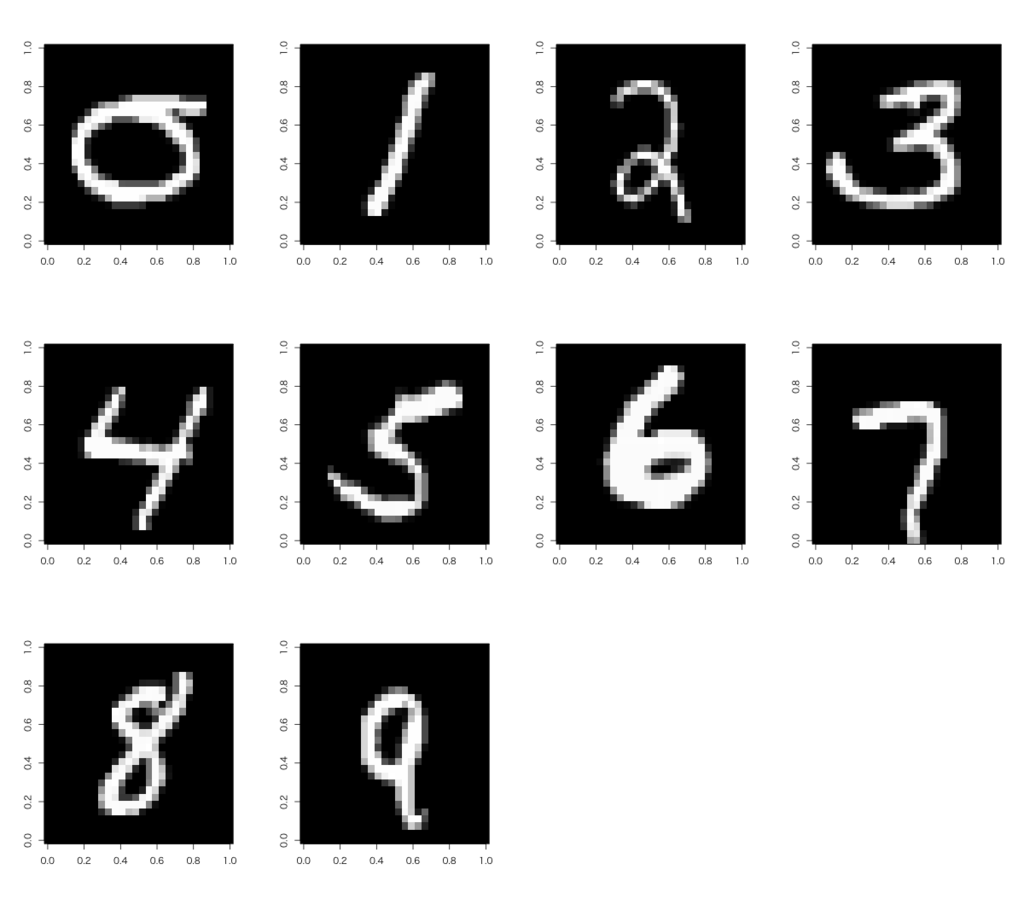I think the participants of MNIST handwritten digits have distinct senses of beauty :P) Actually there are some digits that cannot be identified even by human eyes... but for this reason many attendees of the MNIST competition may get encouraged to identify such difficult digits by super-great machine learning classifiers of their own.

### Xgboost (eXtreme Gradient Boosted Trees)

Xgboost is a growing monster in a lot of machine learning competitions such as Kaggle or KDD Cup. The original one is the gradient boosted trees (GDBT) and Xgboost is an accelerated version of GDBT by DMLC project. Now Xgboost is widely known for its superior performance on many competitions and attracts more and more attention from all over the world. That caused much PV on the blog post below, because this post appears almost at the top when you google "Xgboost" in English :P)

OK, let's try it with the same short version of MNIST as in the case of Random forest. The result below was given by super careful parameter tuning.

> train<-read.csv('https://github.com/ozt-ca/tjo.hatenablog.samples/raw/master/r_samples/public_lib/jp/mnist_reproduced/short_prac_train.csv')

> library(xgboost)
> library(Matrix)
> train.mx<-sparse.model.matrix(label~., train)
> test.mx<-sparse.model.matrix(label~., test)
> dtrain<-xgb.DMatrix(train.mx, label=train$label) > dtest<-xgb.DMatrix(test.mx, label=test$label)
> train.gdbt<-xgb.train(params=list(objective="multi:softmax", num_class=10, eval_metric="mlogloss", eta=0.3, max_depth=5, subsample=1, colsample_bytree=0.5), data=dtrain, nrounds=70, watchlist=list(train=dtrain,test=dtest))
	train-mlogloss:1.439942	test-mlogloss:1.488160
	train-mlogloss:1.083675	test-mlogloss:1.177975
	train-mlogloss:0.854107	test-mlogloss:0.977648
# ... omitted ...
	train-mlogloss:0.004172	test-mlogloss:0.176068
	train-mlogloss:0.004088	test-mlogloss:0.176044
	train-mlogloss:0.004010	test-mlogloss:0.176004
> table(test$label,predict(train.gdbt,newdata=dtest)) 0 1 2 3 4 5 6 7 8 9 0 95 0 0 1 0 0 3 0 1 0 1 0 99 0 0 0 0 0 1 0 0 2 0 0 96 2 0 0 1 1 0 0 3 0 0 1 93 0 0 0 1 2 3 4 0 0 1 1 95 0 1 0 0 2 5 0 1 0 1 0 98 0 0 0 0 6 0 0 1 0 1 2 95 0 1 0 7 0 0 0 0 1 0 0 96 0 3 8 0 4 1 0 1 0 0 0 93 1 9 0 0 0 0 4 1 0 2 0 93 > sum(diag(table(test$label,predict(train.gdbt,newdata=dtest))))/nrow(test)
 0.953 # Accuracy 95.3%


95.3% is better than Random Forest. But what I emphasize here is that it depends on parameter tuning, unlike Random Forest. In particular a random seed may affect the accuracy.

### Deep Learning

Now Deep Learning is a growing monster in "artificial intelligence" industry, not only in machine learning field. For the first time, there was only Deep Neural Network (DNN). But currently we have a lot of variants, such as Convolutional Neural Network (CNN) for image recognition or Recurrent Neural Network (RNN) for sequential datasets like natural language texts, voice speeches or continuous sounds.

As development of Deep Learning expands, although we had Theano or PyLearn2 in the early age, currently we have a lot of user-friendly implementation of Deep Learning such as Torch, Caffe, Chainer, CNTK or TensorFlow so we can very easily try Deep Learning by them.

In this blog, I already showed a simple example with {mxnet} from MXnet so here I only cited an example using CNN with {mxnet} on the short version of MNIST.

Below is a simple example with LeNet, a typical CNN setup.

# Installation
> install.packages("drat", repos="https://cran.rstudio.com")
> install.packages("mxnet")
> library(mxnet)

# Data preparation
> train<-data.matrix(train)
> test<-data.matrix(test)
> train.x<-train[,-1]
> train.y<-train[,1]
> train.x<-t(train.x/255)
> test_org<-test
> test<-test[,-1]
> test<-t(test/255)

> devices <- mx.cpu()
> mx.set.seed(0)

> data <- mx.symbol.Variable("data")
> # first conv
> conv1 <- mx.symbol.Convolution(data=data, kernel=c(5,5), num_filter=20)
> tanh1 <- mx.symbol.Activation(data=conv1, act_type="relu")
> pool1 <- mx.symbol.Pooling(data=tanh1, pool_type="max",
+                            kernel=c(2,2), stride=c(2,2))
> drop1 <- mx.symbol.Dropout(data=pool1,p=0.5)
> # second conv
> conv2 <- mx.symbol.Convolution(data=drop1, kernel=c(5,5), num_filter=50)
> tanh2 <- mx.symbol.Activation(data=conv2, act_type="relu")
> pool2 <- mx.symbol.Pooling(data=tanh2, pool_type="max",
+                            kernel=c(2,2), stride=c(2,2))
> drop2 <- mx.symbol.Dropout(data=pool2,p=0.5)
> # first fullc
> flatten <- mx.symbol.Flatten(data=drop2)
> fc1 <- mx.symbol.FullyConnected(data=flatten, num_hidden=500)
> tanh4 <- mx.symbol.Activation(data=fc1, act_type="relu")
> drop4 <- mx.symbol.Dropout(data=tanh4,p=0.5)
> # second fullc
> fc2 <- mx.symbol.FullyConnected(data=drop4, num_hidden=10)
> # loss
> lenet <- mx.symbol.SoftmaxOutput(data=fc2)
> train.array <- train.x
> dim(train.array) <- c(28, 28, 1, ncol(train.x))
> test.array <- test
> dim(test.array) <- c(28, 28, 1, ncol(test))
> mx.set.seed(0)
> tic <- proc.time()
> model <- mx.model.FeedForward.create(lenet, X=train.array, y=train.y,
+                                      ctx=devices, num.round=60, array.batch.size=100,
+                                      learning.rate=0.05, momentum=0.9, wd=0.00001,
+                                      eval.metric=mx.metric.accuracy,
+                                      epoch.end.callback=mx.callback.log.train.metric(100))
Start training with 1 devices
 Train-accuracy=0.0975510204081633
# omitted #
 Train-accuracy=0.9822
> print(proc.time() - tic)
ユーザ   システム       経過
784.666      3.767    677.921
> preds <- predict(model, test.array)
> pred.label <- max.col(t(preds)) - 1
> table(test_org[,1],pred.label)
pred.label
0   1   2   3   4   5   6   7   8   9
0  99   0   0   0   0   0   1   0   0   0
1   0  99   0   0   1   0   0   0   0   0
2   0   0  98   0   0   0   0   1   1   0
3   0   0   0  98   0   1   0   0   1   0
4   0   2   0   0  97   0   1   0   0   0
5   0   0   0   0   0  99   1   0   0   0
6   0   0   0   0   0   0 100   0   0   0
7   0   0   0   0   0   0   0  99   1   0
8   0   0   0   0   0   0   0   0 100   0
9   0   0   0   0   2   0   0   0   0  98
> sum(diag(table(test_org[,1],pred.label)))/1000
 0.987 # Accuracy 98.7%


Great, CNN by {mxnet} achieved 98.7%, further better than Random Forest or Xgboost.

### Bayesian Modeling with MCMC

This is a strong method for models that are too much complicated to be handled with usual linear models and/or generalized linear models because of excessive random effects or any other individual variance. I think an advantage of Bayesian modeling is its simplicity despite complexity of datasets: even simple and elegant equations can model complex signals.

Below is a simple example of modeling with Stan. In this example, the number of conversion is supposed to be fitted by daily budgets of ads with a hierarchical bayesian including seasonality and 2nd-level differential trend. The former is a Stan code and the latter is an R script with {rstan} below.

data {
int<lower=0> N;
real<lower=0> x1[N];
real<lower=0> x2[N];
real<lower=0> x3[N];
real<lower=0> y[N];
}

parameters {
real wk[N];
real trend[N];
real s_trend;
real s_q;
real s_wk;
real<lower=0> a;
real<lower=0> b;
real<lower=0> c;
real d;
}

model {
real q[N];
real cum_trend[N];
for (i in 7:N)
wk[i]~normal(-wk[i-1]-wk[i-2]-wk[i-3]-wk[i-4]-wk[i-5]-wk[i-6],s_wk); // Seasonality with 7-days cycle (day of week)

for (i in 3:N)
trend[i]~normal(2*trend[i-1]-trend[i-2],s_trend); // 2nd-level differential trend

cum_trend<-trend;
for (i in 2:N)
cum_trend[i]<-cum_trend[i-1]+trend[i];

for (i in 1:N)
q[i]<-y[i]-wk[i]-cum_trend[i]; // Decomposing the dependent variable into a linear model, seasonality and trend
for (i in 1:N)
q[i]~normal(a*x1[i]+b*x2[i]+c*x3[i]+d,s_q); // Sampling for the linear model
}

> d<-read.csv('https://github.com/ozt-ca/tjo.hatenablog.samples/raw/master/r_samples/public_lib/DM_sampledata/example_bayesian_modeling.csv')
> dat<-list(N=nrow(d),y=d$cv,x1=d$ad1,x2=d$ad2,x3=d$ad3)
> library(rstan)
# Parallel computing
> rstan_options(auto_write = TRUE)
> options(mc.cores = parallel::detectCores())
> fit<-stan(file='https://github.com/ozt-ca/tjo.hatenablog.samples/raw/master/r_samples/public_lib/DM_sampledata/hb_trend_cum_wk.stan',data=dat,iter=1000,chains=4)
starting worker pid=4813 on localhost:11406 at 00:03:29.822
starting worker pid=4821 on localhost:11406 at 00:03:30.007
starting worker pid=4829 on localhost:11406 at 00:03:30.188
starting worker pid=4837 on localhost:11406 at 00:03:30.370

SAMPLING FOR MODEL 'hb_trend_cum_wk' NOW (CHAIN 1).

Chain 1, Iteration:   1 / 1000 [  0%]  (Warmup)
SAMPLING FOR MODEL 'hb_trend_cum_wk' NOW (CHAIN 2).

Chain 2, Iteration:   1 / 1000 [  0%]  (Warmup)
SAMPLING FOR MODEL 'hb_trend_cum_wk' NOW (CHAIN 3).

Chain 3, Iteration:   1 / 1000 [  0%]  (Warmup)
SAMPLING FOR MODEL 'hb_trend_cum_wk' NOW (CHAIN 4).

Chain 4, Iteration:   1 / 1000 [  0%]  (Warmup)

# ... omitted ... #

Chain 3, Iteration: 1000 / 1000 [100%]  (Sampling)#
#  Elapsed Time: 34.838 seconds (Warm-up)
#                16.5852 seconds (Sampling)
#                51.4232 seconds (Total)
#

Chain 4, Iteration: 1000 / 1000 [100%]  (Sampling)#
#  Elapsed Time: 42.5642 seconds (Warm-up)
#                46.8373 seconds (Sampling)
#                89.4015 seconds (Total)
#

Chain 2, Iteration: 1000 / 1000 [100%]  (Sampling)#
#  Elapsed Time: 47.8614 seconds (Warm-up)
#                44.052 seconds (Sampling)
#                91.9134 seconds (Total)
#

Chain 1, Iteration: 1000 / 1000 [100%]  (Sampling)#
#  Elapsed Time: 41.7805 seconds (Warm-up)
#                50.8883 seconds (Sampling)
#                92.6688 seconds (Total)
#

# Computing a mode of each parameter from posterior distribution
> fit.smp<-extract(fit)
> dens_a<-density(fit.smp$a) > dens_b<-density(fit.smp$b)
> dens_c<-density(fit.smp$c) > dens_d<-density(fit.smp$d)
> a_est<-dens_a$x[dens_a$y==max(dens_a$y)] > b_est<-dens_b$x[dens_b$y==max(dens_b$y)]
> c_est<-dens_c$x[dens_c$y==max(dens_c$y)] > d_est<-dens_d$x[dens_d$y==max(dens_d$y)]
> trend_est<-rep(0,100)
> for (i in 1:100) {
+ tmp<-density(fit.smp$trend[,i]) + trend_est[i]<-tmp$x[tmp$y==max(tmp$y)]
+ }
> week_est<-rep(0,100)
> for (i in 1:100) {
+ tmp<-density(fit.smp$wk[,i]) + week_est[i]<-tmp$x[tmp$y==max(tmp$y)]
+ }
> pred<-a_est*d$ad1+b_est*d$ad2+c_est*d$ad3+d_est+cumsum(trend_est)+week_est > matplot(cbind(d$cv,pred,d_est+cumsum(trend_est)),type='l',lty=1,lwd=c(2,3,2),col=c('black','red','#008000'),ylab="CV")
> legend("topleft",c("Data","Predicted","Trend"),col=c('black','red','#008000'),lty=c(1,1),lwd=c(2,3,2),cex=1.2)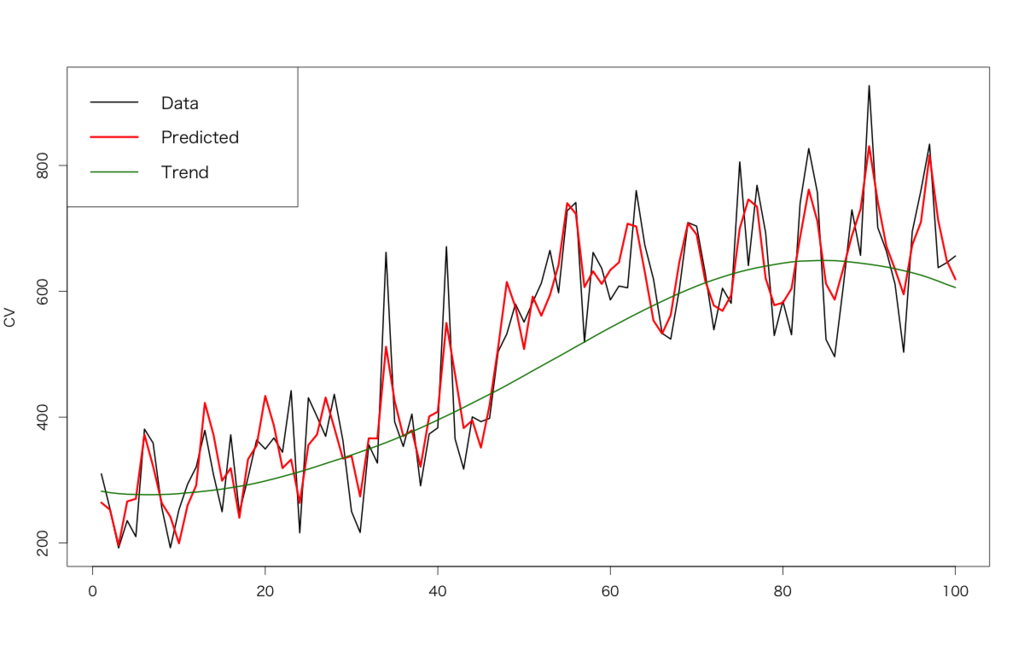Even without any prior knowledge and just with adding hierarchical effect of seasonality and 2nd-level differential trend, this model successfully modeled the number of daily conversion.

### word2vec

I think word2vec is a growing sun in the field of natural language processing since 2013. This is a method that can express a similarity between words and even summation / differentiation of meanings of words. These points are useful for preprocessing of NLP datasets or arranging feature vectors.

For implementation of word2vec, gensim would be the best I think. It can be installed via easy_install. Please don't forget to install also Cython in order to speed up gensim package.

$easy_install -U gensim$ pip install cython


The original dataset is cited from Chapter 10-12 of "The Principles of Psychology" of William James*3, publicly open on the web. After deleting some stop words by Vim and saving it as 'wjames.txt' , let's run as below in Python.

>>> from gensim.models import word2vec
>>> data = word2vec.Text8Corpus('wjames.txt')
>>> model = word2vec.Word2Vec(data, size=200, window=10)
>>> out = model.most_similar(positive=[u'attention'])
>>> for x in out:
...     print x,x
...
their 0.999979555607
may 0.999979436398
in 0.999977529049
would 0.999976277351
them 0.999974668026
be 0.999974489212
whether 0.999972045422
not 0.999970495701
even 0.999967932701
effort 0.999967455864

>>> out = model.most_similar(positive=[u'mind'])
>>> for x in out:
...     print x,x
...
which 0.99997907877
will 0.999977886677
In 0.999977707863
an 0.999976992607
from 0.999976754189
We 0.999976754189
these 0.999976634979
it 0.999976634979
its 0.999975919724
must 0.999974668026

>>> out = model.most_similar(positive=[u'consciousness'])
>>> for x in out:
...     print x,x
...
It 0.999976396561
something 0.999970972538
like 0.999970674515
there 0.999970674515
me 0.999969422817
without 0.999968230724
some 0.999967932701
itself 0.999967575073
my 0.999966204166
now 0.999965965748

>>> out = model.most_similar(positive=[u'attention',u'consciousness'])
>>> for x in out:
...     print x,x
...
its 0.999987006187
an 0.999986886978
In 0.999986767769
will 0.99998664856
it 0.999986588955
from 0.99998652935
these 0.999985694885
which 0.999985158443
on 0.999984562397
most 0.999984145164

>>> out = model.most_similar(positive=[u'attention'],negative=[u'consciousness'])
>>> for x in out:
...     print x,x
...
clothes 0.0337831713259
grounds 0.030175793916
purposes 0.030147626996
conceptual 0.0281115546823
outset 0.025294739753
known. 0.0231171734631
minimum 0.0180826820433
remained 0.0178120266646
habit 0.0177181772888
force. 0.017620716244


"Attention minus Consciousness" mean clothes, grounds, purposes, conceptual, outset, habit or force... ??? :P) This simple result looks even interesting.

### K-means Clustering

Clustering is a typical method in unsupervised learning. There are a lot of variants of clustering and indeed we can choose from a wide range of implementation in various programming language, so here I try K-means clustering because it's widely used and the simplest among clustering methods. This sample dataset is from my own book which contains revenue of books, cloths, cosmetics, foods and liquors.

> d<-read.csv('https://github.com/ozt-ca/tjo.hatenablog.samples/raw/master/r_samples/public_lib/DM_sampledata/ch5_3.txt',header=T,sep=' ')
> d.km<-kmeans(d,centers=3)
> d1<-cbind(d,d.km$cluster) > names(d1)<-'cluster' > res<-with(d1,aggregate(d1[,-6],list(cluster=cluster),mean)) # Summarizing a result of clustering > res cluster books cloths cosmetics foods liquors 1 1 9.047619 13.57143 5.285714 4.333333 7.571429 2 2 46.060606 11.36364 4.575758 5.090909 5.242424 3 3 28.739130 10.28261 4.478261 5.043478 6.043478 > barplot(as.matrix(res[order(res$books),-6]),col=rainbow(5))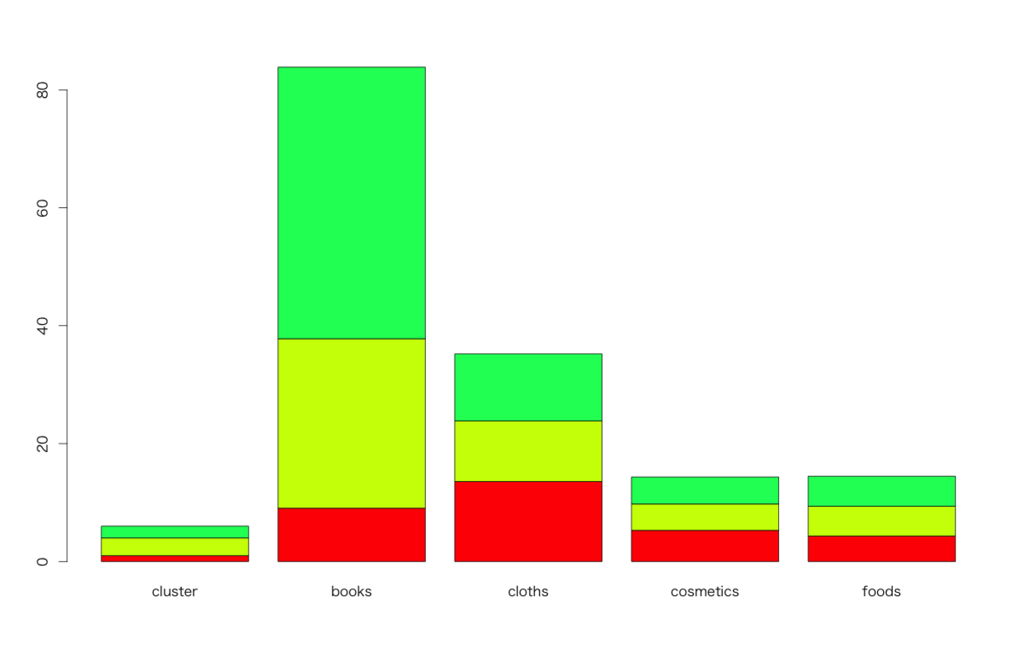This is a very rough visualization. Each color means each cluster given by K-means and its area indicates a ratio of 3 clusters in each purchase domain. In actual business use, for example, K-means can be used for a 3-steps procedure: first K-means clustering categorizes users into some clusters, second a supervised learning classifier is trained based on the labels that is given by K-means, and finally the trained classifier predicts labels of newly acquired users.

### Graph Theory & Network Analysis

These days I found there may be a lot of datasets that can be represented by edge list format and/or Markov chain in various field / industry, so now I'm working a little hard to learn graph theory and network analysis.

Here I tried it on a famous "Karate" dataset*4 just as an easy example of typical data mining methods in graph theory and network analysis. Using {igraph} package, I computed betweenness centrality for each node (person) as a strength as "hub of human relationship" role and drew its graph with Fruchterman-Reingold algorithm with which a node is placed nearer to the other node if both are more strongly related. Using {linkcomm} package, I computed communities under an assumption that one person can be categorized into multiple communities and drew its graph.

> library(igraph)
> g<-graph.edgelist(as.matrix(karate),directed=F)
> g
IGRAPH U--- 34 78 --
+ edges:
  1-- 2  1-- 3  2-- 3  1-- 4  2-- 4  3-- 4  1-- 5  1-- 6  1-- 7  5-- 7  6-- 7  1-- 8  2-- 8  3-- 8  4-- 8
  1-- 9  3-- 9  3--10  1--11  5--11  6--11  1--12  1--13  4--13  1--14  2--14  3--14  4--14  6--17  7--17
  1--18  2--18  1--20  2--20  1--22  2--22 24--26 25--26  3--28 24--28 25--28  3--29 24--30 27--30  2--31
  9--31  1--32 25--32 26--32 29--32  3--33  9--33 15--33 16--33 19--33 21--33 23--33 24--33 30--33 31--33
 32--33  9--34 10--34 14--34 15--34 16--34 19--34 20--34 21--34 23--34 24--34 27--34 28--34 29--34 30--34
 31--34 32--34 33--34
> g.bw<-betweenness(g) # Computing betweenness centrality
> g.ocg<-getOCG.clusters(karate) # Estimating OCG clusters
> par(mfrow=c(1,2))
> plot(g,vertex.size=g.bw/10,layout=layout.fruchterman.reingold)
> plot(g.ocg,type='graph',layout=layout.fruchterman.reingold)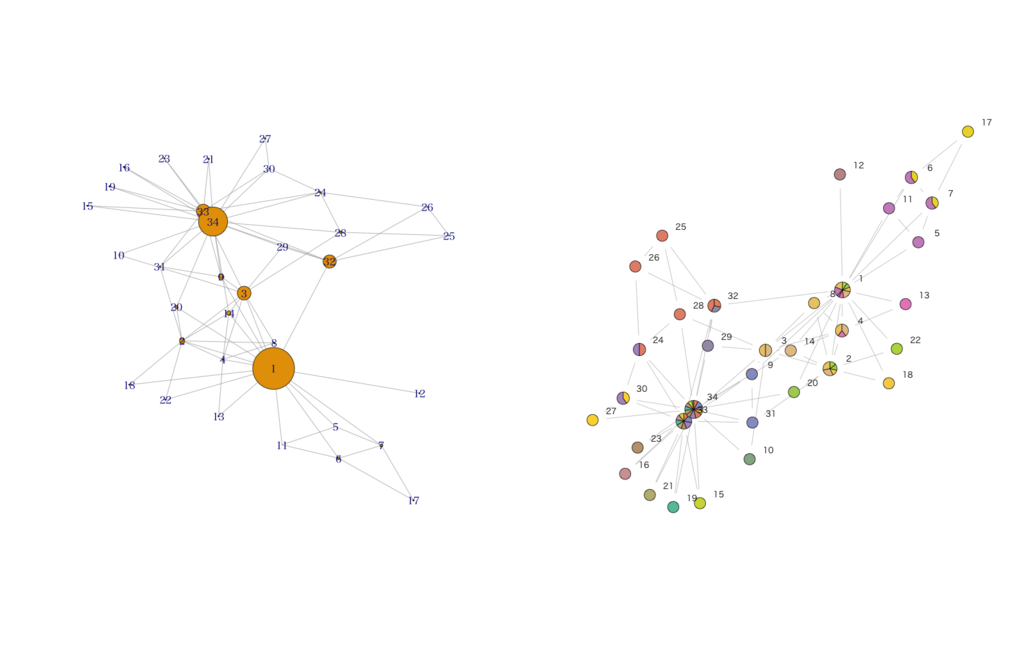#1 and #34 look "bosses" of two major groups, #33 and #34 may be their subordinates, and #3 and #32 appear to be "mediators" for the two major groups.

### Other useful methods

Actually I don't run these two methods below by my own hand for actual works :P), although they are widely used in data science industry. So examples below may not be practical and less useful...

#### Latent Dirichlet Allocation & Topic Modeling

I've been familiar with LDA (Latent Dirichlet Allocation), which is one of the representative methods of topic modeling, because I asked junior members of my team in my previous job to implement it. Roughly speaking, topic modeling is a method to estimate each probability of each word (in a corpus) in a document that can be clustered as a certain topic from many documents. Indeed it's widely used for documents categorization, but it can be also used for any other dataset than documents, in which the dataset is composed with some samples similar to language words.

in general, LDA is implemented as a component of an automated system, so it's easy to get libraries or packages in Python, rather than R. Indeed gensim (see above for word2vec) also contains implementation of LDA. However, there is {lda} package even in R and we can try it quickly on R environment. Below is a quick example cited from a post by id:MikuHatsune.

FYI I changed the dataset to 'newsgroups'. This is a dataset enclosed in {lda} package like 'cora', that contains 20,000 articles with 20 categories from the newsgroup on the Internet in the early age.

> library(lda)
> data(newsgroup.train.documents)
> data(newsgroup.vocab)
# First 10 word frequency of the first article
V1 V2
1    archive  4
2       name  2
3    atheism 10
4  resources  4
5        alt  2
6       last  1
7   modified  1
8   december  1
9    version  3
10   atheist  9

# Training a topic model
> K <- 20
> result <- lda.collapsed.gibbs.sampler(newsgroup.train.documents, K, newsgroup.vocab, 25, 0.1, 0.1, compute.log.likelihood=TRUE)

# Showed 3 or 20 words composing each topic
> top.words3 <- top.topic.words(result$topics, 3, by.score=TRUE) > top.words20 <- top.topic.words(result$topics, 20, by.score=TRUE)
> top.words3
[,1]   [,2]    [,3]    [,4]    [,5]   [,6]   [,7]     [,8]  [,9]     [,10]     [,11]  [,12]      [,13]
[1,] "he"   "god"   "space" "drive" "that" "that" "window" "it"  "that"   "com"     "he"   "windows"  "the"
[2,] "they" "that"  "the"   "scsi"  "it"   "was"  "file"   "car" "israel" "medical" "team" "software" "of"
[3,] "was"  "jesus" "of"    "mb"    "mr"   "of"   "db"     "you" "not"    "was"     "game" "graphics" "and"
[,14]        [,15]  [,16]  [,17]  [,18]    [,19]   [,20]
[1,] "key"        "god"  "you"  "you"  "edu"    "space" "that"
[2,] "encryption" "that" "that" "it"   "com"    "nasa"  "we"
[3,] "chip"       "of"   "gun"  "they" "writes" "for"   "you"
> top.words20
[,1]        [,2]        [,3]         [,4]         [,5]             [,6]      [,7]          [,8]
[1,] "he"        "god"       "space"      "drive"      "that"           "that"    "window"      "it"
[2,] "they"      "that"      "the"        "scsi"       "it"             "was"     "file"        "car"
[3,] "was"       "jesus"     "of"         "mb"         "mr"             "of"      "db"          "you"
[4,] "were"      "he"        "nasa"       "card"       "stephanopoulos" "not"     "server"      "my"
[5,] "she"       "the"       "and"        "disk"       "president"      "it"      "motif"       "that"
[6,] "had"       "of"        "launch"     "output"     "you"            "as"      "widget"      "use"
[7,] "and"       "is"        "satellite"  "file"       "we"             "you"     "mit"         "or"
[8,] "we"        "not"       "center"     "controller" "he"             "writes"  "sun"         "com"
[9,] "that"      "we"        "orbit"      "entry"      "government"     "drugs"   "uk"          "driver"
[10,] "her"       "his"       "lunar"      "drives"     "is"             "are"     "com"         "engine"
[11,] "armenians" "you"       "in"         "ide"        "not"            "were"    "edu"         "cars"
[12,] "his"       "bible"     "gov"        "mhz"        "this"           "article" "display"     "if"
[13,] "turkish"   "people"    "earth"      "bus"        "jobs"           "who"     "set"         "wiring"
[14,] "the"       "christian" "by"         "system"     "what"           "in"      "application" "get"
[15,] "there"     "armenian"  "to"         "memory"     "com"            "about"   "windows"     "but"
[16,] "said"      "it"        "spacecraft" "mac"        "clinton"        "sex"     "code"        "oil"
[17,] "armenian"  "who"       "mission"    "dos"        "if"             "greek"   "lib"         "me"
[18,] "him"       "christ"    "south"      "if"         "believe"        "people"  "cs"          "can"
[19,] "me"        "turkish"   "on"         "windows"    "think"          "is"      "tar"         "up"
[20,] "went"      "are"       "mars"       "pc"         "people"         "livesey" "xterm"       "speed"
[,9]      [,10]        [,11]      [,12]         [,13]        [,14]        [,15]        [,16]
[1,] "that"    "com"        "he"       "windows"     "the"        "key"        "god"        "you"
[2,] "israel"  "medical"    "team"     "software"    "of"         "encryption" "that"       "that"
[3,] "not"     "was"        "game"     "graphics"    "and"        "chip"       "of"         "gun"
[4,] "you"     "in"         "season"   "image"       "government" "clipper"    "is"         "the"
[5,] "israeli" "disease"    "games"    "dos"         "israel"     "keys"       "it"         "he"
[6,] "to"      "msg"        "hockey"   "ftp"         "jews"       "to"         "not"        "guns"
[7,] "of"      "aids"       "play"     "files"       "militia"    "government" "we"         "we"
[8,] "is"      "patients"   "players"  "version"     "states"     "privacy"    "to"         "they"
[9,] "people"  "article"    "year"     "file"        "their"      "security"   "you"        "not"
[10,] "jews"    "writes"     "his"      "available"   "by"         "escrow"     "believe"    "have"
[11,] "who"     "use"        "league"   "pub"         "united"     "secure"     "jesus"      "is"
[12,] "are"     "food"       "teams"    "mail"        "state"      "nsa"        "evidence"   "if"
[13,] "their"   "it"         "baseball" "information" "congress"   "des"        "he"         "your"
[14,] "they"    "apr"        "win"      "pc"          "turkey"     "law"        "there"      "people"
[15,] "war"     "hiv"        "nhl"      "system"      "amendment"  "the"        "christians" "weapons"
[16,] "it"      "university" "player"   "anonymous"   "um"         "system"     "his"        "it"
[17,] "we"      "edu"        "was"      "for"         "law"        "will"       "christian"  "are"
[18,] "arab"    "had"        "vs"       "mac"         "mr"         "public"     "they"       "of"
[19,] "human"   "health"     "pts"      "data"        "arms"       "of"         "do"         "was"
[20,] "were"    "my"         "division" "internet"    "turks"      "pgp"        "atheism"    "do"
[,17]     [,18]     [,19]      [,20]
[1,] "you"     "edu"     "space"    "that"
[2,] "it"      "com"     "nasa"     "we"
[3,] "they"    "writes"  "for"      "you"
[4,] "is"      "article" "program"  "is"
[5,] "that"    "it"      "edu"      "to"
[6,] "writes"  "my"      "the"      "it"
[7,] "edu"     "apr"     "email"    "they"
[8,] "don"     "you"     "flight"   "do"
[9,] "uiuc"    "bike"    "system"   "not"
[10,] "think"   "car"     "moon"     "my"
[11,] "your"    "cs"      "henry"    "he"
[12,] "article" "dod"     "research" "have"
[13,] "not"     "ca"      "engine"   "people"
[14,] "my"      "pitt"    "model"    "what"
[15,] "com"     "cars"    "sale"     "church"
[16,] "would"   "too"     "send"     "be"
[17,] "me"      "like"    "shuttle"  "think"
[18,] "if"      "ride"    "you"      "can"
[19,] "do"      "ac"      "entries"  "there"
[20,] "cso"     "uucp"    "looking"  "if"

# Just checked the first 5 articles
> N <- 5
> topic.proportions <- t(result$document_sums) / colSums(result$document_sums)
> topic.proportions <- topic.proportions[1:N, ]
> topic.proportions[is.na(topic.proportions)] <-  1 / K
> colnames(topic.proportions) <- apply(top.words3, 2, paste, collapse=" ")
>
> par(mar=c(5, 14, 2, 2))
> barplot(topic.proportions, beside=TRUE, horiz=TRUE, las=1, xlab="proportion")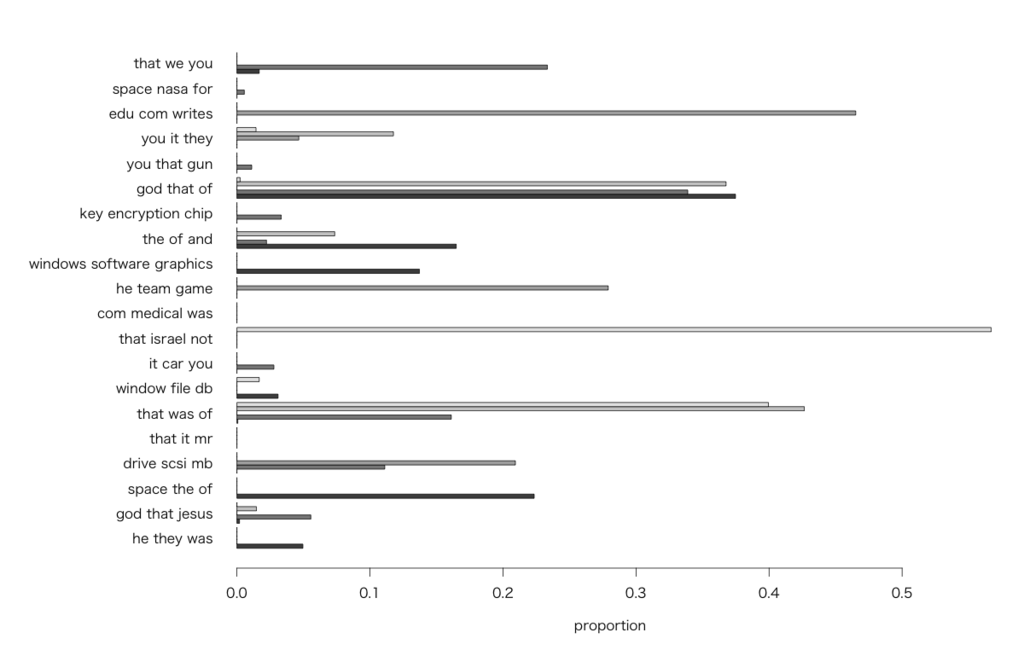The last part showed a plot of probability for each topic on the first 5 articles. It indicates some topics contain some specific articles and so on. About this sample script, 'newsgroup' dataset is divided into train / test sets, so you can build a model with the training dataset and check it with the test dataset.

#### Factorization (SVD, NMF)

This is also a widely used methodology in data science industry and indeed junior members of my team implemented it and even my colleagues loved to read about it in the journal club in my previous job so I'm much familiar with it, although I've never tried by my own hand :P) Factorization is one of the most important bases of recommendation, so many data science guys appear to love it.

In essence, factorization is merely a dimension reduction, i.e. removing unnecessary components from the dataset. However, it enables us to compute recommendation scores easily from even sparse datasets. Unfortunately my knowledge is still not enough, so here I cited some example scripts from the blogs of id:SAM and id:a_bicky.

# Simulated dataset of Chapter 9 in my own book

# SVD reducing rank to 4
> res.svd <- svd(M) # SVD
> u <- res.svd$u > v <- res.svd$v
> d <- diag(res.svd\$d)
> d_r <- d
> for (i in 5:11) {
+     d_r[i, i] <- 0
+ }
> R_svd <- as.matrix(M) %*% v %*% solve(d) %*% d_r %*% t(v)
> colnames(R_svd) <- colnames(M)
> head(round(R_svd, 2)) # Recommendation scores
book cosmetics electronics food imported liquor magazine  sake stationery  toy travel
[1,] 0.74      1.21        0.15 0.36     0.24   1.00     0.82  0.14       0.42 1.07   0.19
[2,] 0.70      0.07        0.27 0.64     0.91  -0.02    -0.06 -0.03       0.63 0.02   0.23
[3,] 1.12      0.71        0.34 0.73     0.14   0.95     0.40  1.04       0.72 1.04   0.38
[4,] 1.40      0.68        0.45 1.01     0.72   0.92     0.33  0.79       1.00 1.02   0.47
[5,] 0.81      0.06        0.27 0.52    -0.03   0.96    -0.05  0.99       0.51 1.02   0.30
[6,] 1.13      1.17        0.35 0.87     0.20  -0.05     0.76  1.12       0.78 0.03   0.40

# NMF reducing rank to 4
> library(NMF)
> res.nmf <- nmf(M, 4, seed=1234) # NMF
> w <- basis(res.nmf)
> h <- coef(res.nmf)
> h_z <- rbind(h, rep(0, 11))
> R_nmf <- w %*% h
> head(round(R_nmf, 2)) # Recommendation scores
book cosmetics electronics food imported liquor magazine sake stationery  toy travel
[1,] 0.81      1.36        0.00 0.52     0.00   0.97     0.68 0.00       0.54 1.13   0.00
[2,] 0.64      0.00        0.00 0.48     0.88   0.00     0.00 0.00       0.47 0.00   0.52
[3,] 1.07      0.75        0.47 0.70     0.00   1.00     0.37 0.76       0.71 1.17   0.00
[4,] 1.57      0.61        0.33 1.10     1.02   0.85     0.30 0.53       1.10 0.99   0.61
[5,] 0.78      0.00        0.44 0.50     0.00   0.95     0.00 0.70       0.52 1.11   0.00
[6,] 1.19      1.38        0.81 0.85     0.00   0.00     0.68 1.30       0.79 0.00   0.00


You'll find that recommendation scores of SVD and NMF for the first 6 users were different from each other, i.e. the former contains some negative values and the latter doesn't. Of course actual implementations are further complicated because some tunings or computational costs should be considered in practice.

### My own thoughts on difference between statistics and machine learning

I think statistics and machine learning can be distinguished as below:

• Statistics values "interpretability"
• Machine Learning values "predictivity"

In general, people tend to emphasize "interpretable" points such as magnitude of parameters (regression coefficients) when linear models or GLMs are used in terms of statistics. On the other hand, people tend to emphasize "predictivity" of models for test (future) datasets in terms of machine learning. Understanding this difference is very important. For example, it affects how to evaluate models. In terms of statistics, static indices such as AIC or BIC are used to evaluate models. However, in terms of machine learning, various kinds of cross validation are widely used to evaluate ones and in particular "generalization" can be a first priority.

Understanding what kind of aspects affects performance of models is also important. In principle, statistical models (such as linear models or GLMS) are mainly affected by choice of independent variables, but machine learning models (Random Forest or Deep Learning) are influenced by rather model parameters and its tuning*5 than choice of independent variables. In case of machine learning, grid searching for parameter tuning can be the most important.

Thus we have to choose entirely different ways to improve performance of models if we choose a specific modeling method from either statistics or machine learning. Conversely, from my own personal experience, if we choose the most appropriate modeling method (even if it's just a logistic regression) for a specific issue, we'll get excellent performance without high-level and advanced methods such as Xgboost or Deep Learning.

### FYI

Actually this post is just a translation of a post in my Japanese blog :p)

*1:Actually a concept and framework of hypothesis testing is very much complicated and still there are controversies about whether we can really conclude that one is faster than the other, in particular if issues on effect size, sample size or file drawer problem are involved. However, I think we can conclude so just as "users" of statistics

*2:But in this case you have to consider the number of page views as an "offset" factor

*3:These parts of the book was a matter of my own study when I was a neuroscientist...

*4:An undirected graph dataset about relationships of a Karate club

*5:e.g. the number of hidden layers and the number of units in each layer for Deep Learning